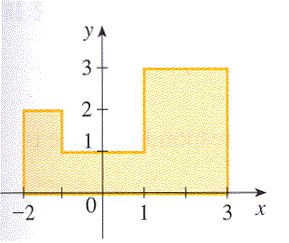Find the centroid of the region shown, not by integration, but by locating the centroids of rectangles and triangles and using additivity of moments.

Hint: It can be shown that the centroid of a triangle is located at the point of intersection of the medians. A median is a line segment from the vertex of a triangle to the opposite side. Recall that the medians intersect at a point two-thirds of the way from each vertex (along the median) to the opposite side.$(\bar{x},\bar{y}) =$ (, )

You can earn partial credit on this problem.